# Electronics and Communication Engineering - Exam Questions Papers

16.

VL = 100 cos (10t + 30°)
R = 10 Ω XL = j10 Ω
XC = - j4 Ω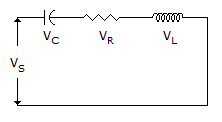Find Vs

 A. 116.57 sin (10t - 29°) V B. 116.57 sin (10t + 29°) V C. 117.00 sin (10t - 29°) V D. 117.00 sin (10t + 29°) V

Explanation: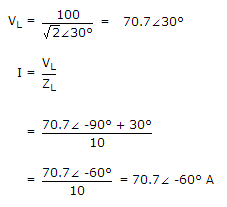VC = XC X I = 7.07 X 4∠ -90° - 60° = 28.28∠ - 150°V

VR = I X R = 7.07 ∠-60° x 10 = 70.7∠ - 60°

Vs = 70.7∠30° + 70.7∠-60° + 28.28∠-150° = 82.44∠-29° V

vs = 116.57 sin (10t - 29°) V.

17.

4 point DFT for x(n) = {2, - 1, 2, 3} is

 A. {6, 4 - 2j, 2, 2j} B. {6, 4j, 2, - 4j} C. {6, -1 + 3j, 4 - 4j, 4 - 2} D. {6, 2j, 4 - 2j, - 2 + 2j}

Explanation:

1 point DFT

X(k) = [W][x(n)]1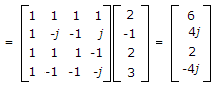18.

Determine the voltage VCB in the circuit below: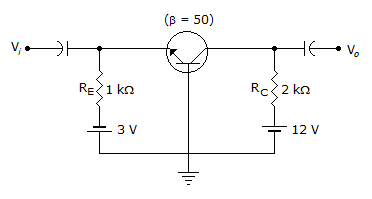A. 3.4 V B. 5.4 V C. 7.4 V D. 9.4 V

Explanation: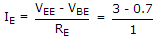= 2.3 mA ≈ IC

VCB = CCC - ICRC = 12 - 2.3 x 2 = 7.4 V.

19.

In a series LCR circuit the maximum inductor voltage is twice the maximum capacitor voltage however current lags by 30° and drop across the inductance is vL = 100 sin 377t V. Assuming the resistance being 20 Ω, Find the values of the capacitance and inductance.

 A. 235 μF, 61.18 μH B. 230 μF, 61.18 μH C. 61.18 μF, 230 μH D. None of the above

Explanation:

vL max = 100 V = ωL Imax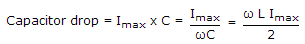2Imax = ωL X ωC Imax

ω2LC = 2

ω2LC = 2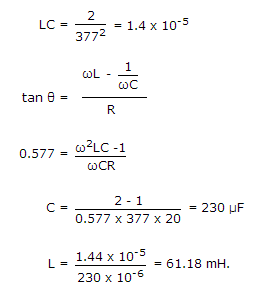20.

In 8085 microprocessor based system running at 3 MHz clock frequency what should be the minimum pulse width of the INTR signal, so that it is recognized successfully?

 A. 0.33 μs B. 0.666 μs C. 5.8 μs D. none of these

Explanation:

T =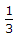x 106 = 0.333 μs

8085 checks INTR, one clock period before the last T-state of an instruction cycle. In 8085, CALL instruction requires 18 T-states.

INTR pulse should be high at least for 17.5 T-states

i.e. for 17.5 x 0.33 x 10-6 = 5.8 μs long.